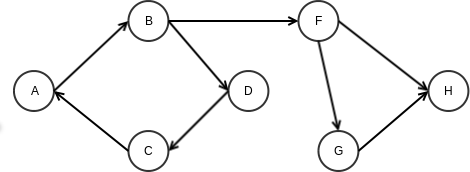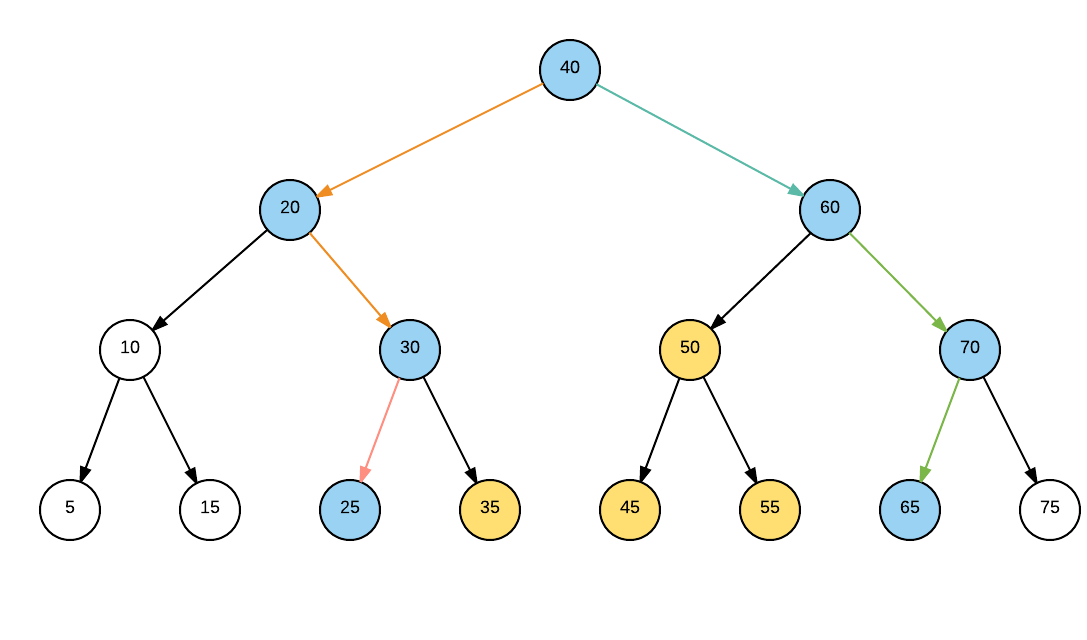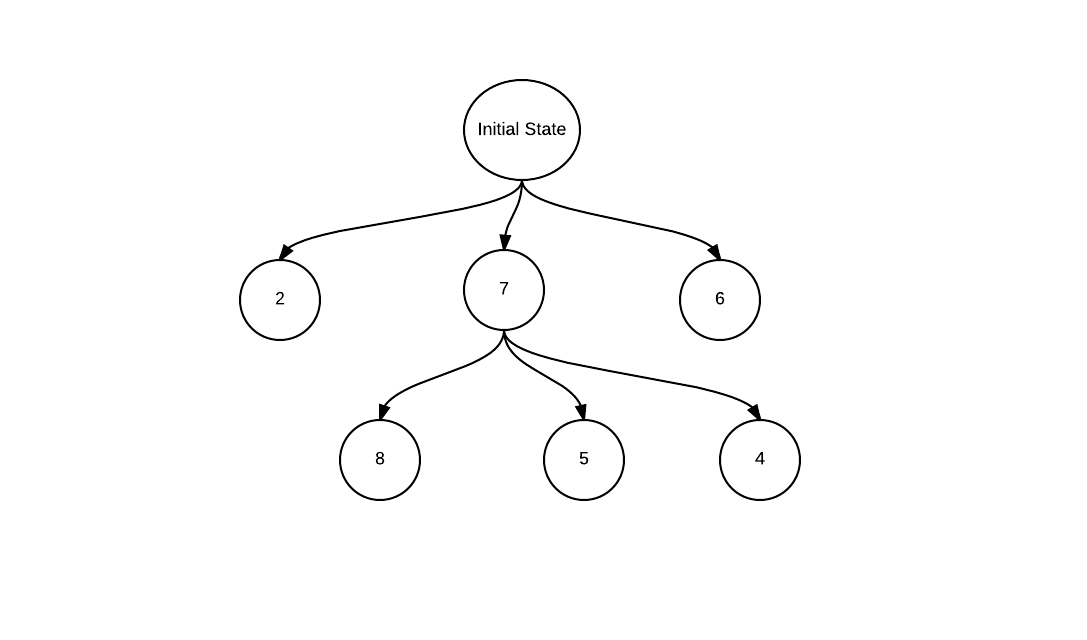# Find Longest Common Prefix

Solving any problem requires one to analyze various available solutions and then look at factors like space complexity, time complexity, and ease of development.

Problem statement: Find the longest common prefix from N number of Strings.

Example: “kamal”, “kamalmeet”, “kamaljeet” should return “kamal”

## Solution 1- Vertical Scanning

Approach: Start with the first element of each string and compare for equality. Continue till a point we do not find the same character.

Pseudo code:

```-- for i=0 to n, of the length of the first (any) string
---- check if ith character is equal for all string
------ if not, return string from 0 to i-1 character```

Code:

``````    public String longestCommonPrefix(String[] strs) {
for (int index = 0; index < strs.length(); index++) {
char ch = strs.charAt(index);
for (String st : strs) {
// one of the strings has ended or char mismatch found
if (index >= st.length() || st.charAt(index) != ch) {
return strs.substring(0, index);
}
}
}
// if you reached here, first string is longest common prefix
return strs;
}``````

Time Complexity: O(S) for Worst case all strings are equal, and S is the sum of all string lengths

Space Complexity: O(1) no additional data structure

## Solution 2 – Horizontal Scanning

Approach: Start by comparing the first two strings and find the longest common prefix. Use this as input and compare it with next string and so on.

Pseudo code:

```-- longestprefix = str
-- for strings in i=1 to N
---- longestprefix = findlongestprefix(longestprefix, str[i])
-- return longestprefix```

Time Complexity: O(S)

Space complexity: O(1)

## Solution 3- Divide and Conquer

Approach: Break down the array of strings into two equal parts, solve for the two subarrays, and find a solution for two results (repeat the process at each step).

# Finding the cycle node in a list

We will need to break the problem into 2 parts, first find if a cycle is available in the list, second where does the cycle starts.

Finding the cycle can be done using tortoise and hare algorithm where we have two pointers in the start. first pointer will move at a speed of one node at a time and second pointer moves at a speed of 2. If these two pointers meet a point, we know there is a cycle.

Next step is to get count of elements in the cycle, which is easy after finding any node in cycle as we did using tortoise and hare algorithm. All we need to do is to take the node we found in the cycle, and move to next until we reach back to original node.

Last step, is to reposition two pointers at start. Next make one of the pointers get a lead of nodes equal to cycle size. Finally start moving the two pointers at a speed of 1, remember one pointer started from start node and second started at Nth node, where N is size of cycle. At the point where these meet is the node where the cycle starts.

```public ListNode detectCycle(ListNode a) {
ListNode pt1=a;
ListNode pt2=a.next;
while(pt1!=pt2 && pt1.next!=null && pt2.next!=null && pt2.next.next!=null) {
pt1=pt1.next;
pt2=pt2.next.next;
}
if(pt1.next==null || pt2.next==null || pt2.next.next==null) return null;
//we have a cycle, lets check number of nodes
ListNode l = pt1;
int count =0;
pt1=pt1.next;
while(pt1!=l) {
count++;
pt1=pt1.next;
}
count++;
pt1=a;
pt2=a;
for(int i=0;i
```

# Chebyshev Distance

Chebyshev distance is an interesting concept stating the relative distance in terms of matrix. For example, if we consider center of a 2-D matrix as starting point

2 2 2 2 2
2 1 1 1 2
2 1 X 1 2
2 1 1 1 2
2 2 2 2 2

Where X is starting point.

On a 2-D plane this distance is max(mod(x2-x1),mod(y2-y1))
or in a 2-D matrix, considering above example,
max(mod( rowindex – maxnum), mod(colindex – maxnum))
maxnum being 2 in above example

A small code to calculate above

public ArrayList<ArrayList> chebyshev(int A) {
ArrayList<ArrayList> arr =new ArrayList<>();
// maxnum = A
//max(mod( rowindex – maxnum), mod(colindex-maxnum))
int size=A*2+1;
int maxnum=A;
for(int i=0;i< size;i++)

{
ArrayList arrin=new ArrayList<>();
for(int j=0;j< size;j++)

{
int rownum=i-maxnum;
//mod
if(rownum<0)rownum*=-1;
int colnum=j-maxnum;
if(colnum<0)colnum*=-1;
//find max
int num=(colnum<rownum)?rownum:colnum;
}
}
return arr;
}

# HashMap- Collision handling using chaining and open addressing

A HashMap is a datastructure which links a key to the value. For example key can be employee id and value might be employee details. The definition actually is true for any map, a hash map adds the functionality of hashing to a simple key-value map. A hash function maps a key to a particular bucket (we can think of it as array position) to add value. For example, if employee id is unique, a good hash function would simply return employee id itself as key. But in case, we are trying to use employee name as key, we might need a better hashing function to generate a bucket id based on employee name.

As we can see from above example, if we do not choose a good hash function, we might not be able to get unique bucket ids for every key. So multiple keys might actually return same bucket id. This causes a collision.

There are 2 important ways to handle collisions

Separate Chaining: In this technique, we create a list inside each bucket to store the objects in case one bucket needs to handle multiple objects. For example, say keys Dave and David returns same hash say bucket number 4 (or array index 4), we would create a linked list in bucket four and store both elements in the list. The advantage is that we can infinitely grow this list (provided no space concerns) and handle any level of collisions.

In this technique, if in case say two keys generate same hash, the first one would be stored at the hash position, and the other one(s) would be stored at next best position. There are again multiple ways to find the next best position. Here are 2 simple ones

Linear Probing: Simply look for next empty space in array. For previous example, say David has a hash value 4, and we found that position 4 is already filled, we will simply look for next empty position, say 5 is empty, we will save David’s object in 5 or if 5 is also booked we will move on to 6 and so on.

Double Hashing: Going to previous example, if we found bucket 4 is full, instead of simply looking for next empty position, we will invoke a new hash function and add the returned number to initial bucket number. Say input David returned 16 from second hash function, we will try to insert the object at 4+16 i.e. 20th position. If 20th position is also filled, we will move to 20+16 i.e. 36 and so on.

Worst case Analysis

The performance of a hashmap usually depends on the hashing function. For example, say we have <=1000 objects and we create a map with 1000 entries. The perfect hashing function would map each entry to a unique entry in the map. In such a case we will have addition, deletion and search at worst case as O(1). Similarly, if we choose a worse hash function, which lets say maps each key to a single bucket (say a dumb hash function return 10, no matter what the input is), we will end up with a worst case search of O(n). Because of the above reason, we cannot guarantee a worst case performance for a hashmap, and hence a Binary Search Tree or a Red Black tree would sometimes be preferred in performance critical operations, as we can guarantee a max search time. Separate Chaining vs Open Addressing An obvious question is that which collision handling technique should be used. Both has its advantages. As a thumb rule, if space is a constraint and we do have an upper bound on number of elements, we can use open addressing. The advantage with Separate chaining is that it can grow indefinitely (if space is available).

# Minimum Priority Queue- Java Implementation

```package com.kamalmeet.www;

/**
* This is a sample implementation of Min Priority queue.
* This will work for any object type which has a valid comparable interface implemented.
*
* @author kamalmeet
*
* @param
*/
public class MinPriorityQueue {

Type minQueue[]=null;
int elements=0;

/**
* Constructor to create a Minimum priority queue of a specific size.
*
* @param size
*/
public MinPriorityQueue(int size){
minQueue=(Type[]) new Object[size];
}

/**
* A new element is always added at the last leaf and then moved up till we find correct position.
*
* @param t
*/
{
if(elements==0)
{
minQueue=t;
System.out.println(minQueue);
elements++;
}
else
{
minQueue[elements]=t;
moveUp(elements);
elements++;

}
}

/**
* Zeroth element is deleted and returned.
* Last element would move to root element and queue would be heapified.
*
* @return
*/
public Type delete()
{
Type min=minQueue;
minQueue=minQueue[--elements];
moveDown(0);
return min;
}

/**
* In Move up or swim method, an element is compared to its parent and moved
* up unless correct position is found.
*
* @param num
*/
public void moveUp(int num)
{
System.out.println("move:"+num);
while(((Comparable)minQueue[num]).compareTo(minQueue[parent(num)])<0)
{
swap(num, parent(num));
num=parent(num);
}
}

/**
* In move down or sink method, an element is compared to its children and moved
* down unless correct position is found.
*
* @param num
*/
public void moveDown(int num)
{
if(leftChild(num)>elements)
return;
Type leftChild=minQueue[leftChild(num)];
if(rightChild(num)>elements)
{
if(((Comparable)leftChild).compareTo(minQueue[num])<0)
{
swap(num, leftChild(num));
return;
}
}
else{
Type rightChild=minQueue[rightChild(num)];
if(((Comparable)leftChild).compareTo(rightChild)<0)
{
if(((Comparable)leftChild).compareTo(minQueue[num])<0)
{
swap(num, leftChild(num));
moveDown(leftChild(num));
}
}
else
{
if(((Comparable)rightChild).compareTo(minQueue[num])<0)
{
swap(num, rightChild(num));
moveDown(rightChild(num));
}
}
}

}

/**
* Method to swap 2 elements.
*
* @param i
* @param j
*/
public void swap(int i, int j)
{
Type t=minQueue[i];
minQueue[i]=minQueue[j];
minQueue[j]=t;
}

/**
* Find parent of an element. usually it is element/2 but we need to take
* care of the fact that array starts from 0.
*
* @param i
* @return
*/
public int parent(int i)
{
if(i==0 || i==1 || i==2)
return 0;
int p=(i+1)/2;
return p-1;
}

/**
* Finds left child for the element.
*
* @param i
* @return
*/
public int leftChild(int i)
{
//as array starts from 0, we will need to add 1 initially and subtract 1 finally
int c=0;
c=(i+1)*2;
return c-1;
}

/**
* Find right child for the element.
*
* @param i
* @return
*/
public int rightChild(int i)
{
//as array starts from 0, we will need to add 1 initially and subtract 1 finally
int c=0;
c=((i+1)*2)+1;
return c-1;
}

/**
* Method to print the minimum queue (for debug).
*/
public void print()
{

for(int i=0;i min= new MinPriorityQueue(10);

min.print();
System.out.println("********************");
System.out.println("deleted:"+min.delete());
min.print();

}

}

```

# Strongly connected components- Kosaraju’s algorithm

Before starting with Kosaraju’s algorithm, you might want to revisit Depth first and beadth first algorithms.

Refer the following graphvertex a,b in a graph are stringly connected if a is reachable from b and b is reachable from a.

In above graph, ABCD subgraph is strongly connected.

Kosaraju’s algorithm helps in finding all such strongly connected components in a graph.

1. Run Depth first search (DFS) on the graph and add verticies on a stack S.
2. Reverse (transpose) the graph by reversing directions of all the edges.
3. Pull a vertex v from Stack S (step 1), run DFS on on vertex on reversed graph created in step 2. All the nodes found in DFS from vertex v are strongly connected. Remove all nodes found in DFS from reversed graph and stack S.

# Find Median of an Array

The simplest way to find median of an array to sort it and find the middle element. But any good sorting algorithm would take N*logN time. Can we do better than that?

Hint: Quick sort.

In quick sort, we choose a random pivot and then place it in the correct sorted position in array, that is all element on left are less than pivot and all elements on right and higher.

Using the same approach we can find median of an array, without actually sorting it.

1. We need to find mid element of the array os size N. Say N=101, we have to find 51st element (for even N, we will need to find N/2 and N/2 + 1).
2. Choose a random pivot (say first element) and find its correct place in array.
3. Now from the two halfs, find the one where mid element from step 1 (51st in this case) will be present. (say first half has 25 element and second has 75, we will discard first half)
4. Repeat from step 2 with current choosen array half.

Worst case performance is N^2. For every element we are dividing one half is halving one or two elements.

Average Case performance is N, as we are discarding N/2 elements in each iteration.

N+N/2+N/4…..

N(1+1/2+1/4…)
N*C (some constant)

# 1-D Range search

Range Search is a very basic alogorithm used in multiple situations, like out of a set of 100 employees, find all whose salary falls between a specific range. As we are talking about sigle aspect (salary), we will use a 1-D range serach approach to solve this problem.

A simple algorithm would use a Balanced binary serach tree, with property that all the nodes in left subtree are smaller than root node and all right subtree node are higher, for any subtree. The balancing property make sure all the root nodes are at a distance on X or X-1, where X is order of lg(N), N being nodes in tree.

Here is an exampleLets say the number represent salary of employess in thousands. And the range we need to find is say 23 and 63. We will traverse tree for both lower and upper bound. Orange arrows signifies traversal for 23 and Green ones show traversal for 63.

This leaves us with three type of nodes.

1. Nodes not traversed and lie out of subrees of traversal path (white nodes)- can be ignored
2. Nodes in path of traversal (blue nodes)- may or may not be in range
3. Node lying between traversal path (Yellow nodes)- these are in range.

Using above information, we can deduce a simple algorithm

1. Input lower range L, and high range H
2. Create a output set S as range set
3. Traverse/ search for L in the tree (if L is smaller than node, move right, else move left)
3a. if the node being traversed is in range, add to set S
3b. If L is less than Node value, Add the whole right subtree of current node to the set S (as it is in range)
4. Traverse/ search for H in the tree (if H is smaller than node, move right, else move left)
4a. If the node being traversed is in range, add to set S
4b. If H is greater than Node value, Add the whole left subtree to the set S (as it is in range)

# A-star Search

While you are playing a game (say rubik’s cube), each state can lead to multiple new states after user makes a move. Or in a graph path finding, one can choose multiple nodes from a given input node. Such situations can be represented by a game tree (graph).Each state can result into multiple new states (will come to the numbers in a moment). The question is, which next state to choose. A* (A-star) Search algorithm can help me make a choice.

We need to understand the following terms before we try to understand the algorithm.

Open Set: Set of moves not evaluated yet.
Closed set: Set of moves already evaluated
Score/ Priority: higher (in some case lower) the score, the closer we are to the solution.

Score can be calculated based on problem we are trying to solve. For example in rubik’s cube, it might be the number of boxes/ rows already matched (say 10 indicates 10 rows of colors matched, will get higher priority than a state with score 7).

Algorithm

1. Define initial state as current state
2. If current state is solution state, return success and exit. Else move on to step 3.
3. Find all states possible from current state, which are not in closed set already and add to open set
4. Add current state to closed set.
5. set an open state with maximum score as current state and remove from open set.
6. Go to 2.

A more elaborate explanation can be found – https://en.wikipedia.org/wiki/A*_search_algorithm
http://web.mit.edu/eranki/www/tutorials/search/

# Initialize a matrix – efficiently

A simple code to initialize a matrix of N*N elements with all same number can be to create 2 for loops from 0 to N and set element to the required number. A silght improvement can be done to this code by

``` for (int i = 0; i < N / 2; i++)
for (int j = i; j < N - i; j++) {
arr[i][j] = 0;
arr[j][i] = 0;
arr[N - 1 - i][N - 1 - j] = 0;
arr[N - 1 - j][N - 1 - i] = 0;
}
```